### Home > GC > Chapter 12 > Lesson 12.2.4 > Problem12-91

12-91.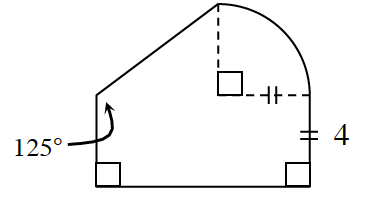Find the area and perimeter of the shape at right. Assume that any non-straight portions of the shape are part of a circle. Show all work.

A right triangle, the first quadrant of a circle, and a rectangle.

Break up the shape into smaller, more manageable shapes.
There is more than one way to do this.

The angle opposite the triangle leg labeled 4 is 35 degrees. The radius of the circle is 4 and the short side of the rectangle is 4.

$125^\circ − 90^\circ = 35^\circ$

Fill in all known angles and lengths.

The other leg in the triangle is labeled, x.

Use trigonometry to find the length of the triangle's unknown leg.

$\tan35^\circ = \frac{4}{x} \text { or } \frac{\sin 55^\circ}{x} = \frac {\sin 35^\circ}{4}$

The long side of the rectangle is labeled x plus 4.

Find the area of each the smaller shapes.

How does each of these smaller areas relate to the area of the larger shape?

The hypotenuse of the triangle is labeled, a. The first quadrant arc of the circle is labeled, b.

Find the lengths of the sides that form the perimeter
the triangle.

Add these answers together to find the perimeter of the entire shape.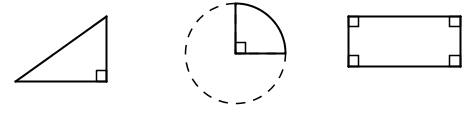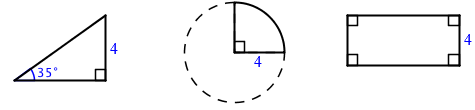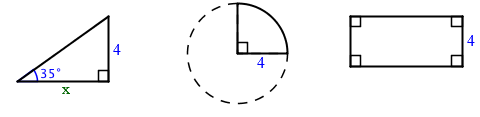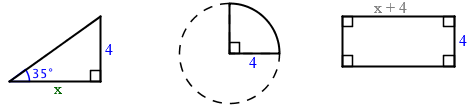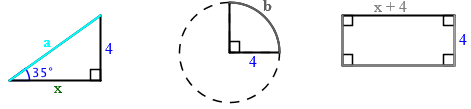$\begin{array}{l} \textit{Area}_{\textit{tri}} = \frac{1}{2} (\text{base}) (\text{height}) \\ \textit{Area}_{\textit{tri}} = \frac{1}{2} (5.71) (4) \\ \textit{Area}_{\textit{tri}} = 11.42 \; \text{units}^2 \end{array}$ $\begin{array}{l} \textit{Area}_{\textit{sec}} = \frac{1}{4} r^2 \pi \\ \textit{Area}_{\textit{sec}} = \frac{1}{4} 4^2 \pi \\ \textit{Area}_{\textit{sec}} \approx 12.566 \; \text{units}^2 \end{array}$ $\begin{array}{l} \textit{Area}_{\textit{rec}} = (\text{base}) (\text{height}) \\ \textit{Area}_{\textit{rec}} = (4) (5.71 + 4) \\ \textit{Area}_{\textit{rec}} = 38.84 \; \text{units}^2 \end{array}$

 $\begin{array}{r} x^2 + 4^2 = a^2 \\ 5.71^2 + 4^2 = a^2 \\ 48.6041 = a^2 \\ 6.972 \approx a \end{array}$ $\begin{array} {l} b = \frac{1}{4} (2 r \pi) \\ b = \frac{1}{4} (2 \cdot 4 \pi) \\ b \approx 6.26 \end{array}$

$\begin{array}{l} \text{Perimeter} = 6.972 + 6.26 + 4 + 9.71 + 4 \\ \qquad \qquad \; \; = 30.942 \; \text{units} \end{array}$

$\text{Area}=62.826\;\text{units}^2$